# Surface Areas and Volume

Surface area and volume are calculated for any three-dimensional geometrical shape. The surface area of any given object is the area or region occupied by the surface of the object. Whereas volume is the amount of space available in an object.

In geometry, there are different shapes and sizes such as sphere, cube, cuboid, cone, cylinder, etc. Each shape has its surface area as well as volume. But in the case of two-dimensional figures like square, circle, rectangle, triangle, etc., we can measure only the area covered by these figures and there is no volume available. Now, let us see the formulas of surface areas and volumes for different 3d-shapes.

## What is Surface Area?

The space occupied by a two-dimensional flat surface is called the area. It is measured in square units. The area occupied by a three-dimensional object by its outer surface is called the surface area. It is also measured in square units.

Generally, Area can be of two types:

(i) Total Surface Area

(ii) Curved Surface Area/Lateral Surface Area

### Total Surface Area

Total surface area refers to the area including the base(s) and the curved part. It is the total of the area covered by the surface of the object. If the shape has a curved surface and base, then the total area will be the sum of the two areas.

### Curved Surface Area/Lateral Surface Area

Curved surface area refers to the area of only the curved part of the shape excluding its base(s). It is also referred to as lateral surface area for shapes such as a cylinder.

## What is Volume?

The amount of space, measured in cubic units, that an object or substance occupies is called volume. Two-dimensional doesn’t have volume but has area only. For example, the Volume of the Circle cannot be found, though the Volume of the sphere can be. It is so because a sphere is a three-dimensional shape.

## Surface Area and Volume Formulas

Below given is the table for calculating Surface area and Volume for the basic geometrical figures:

 Name Perimeter Total Surface Area Curved Surface Area/Lateral Surface Area Volume Figure Square 4b b2 —- —-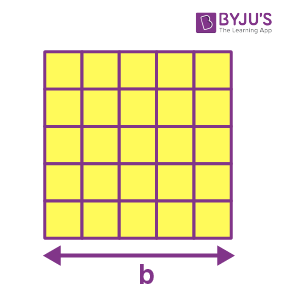Rectangle 2(w+h) w.h —- —-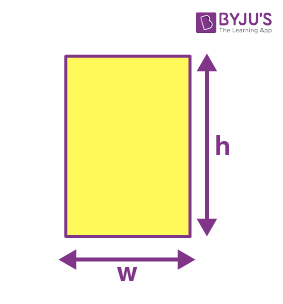Parallelogram 2(a+b) b.h —- —-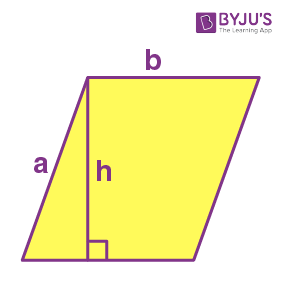Trapezoid a+b+c+d 1/2(a+b).h —- —-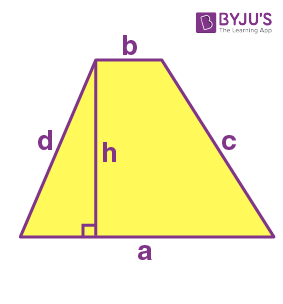Circle 2 π r π r2 —- —-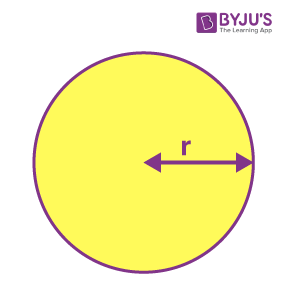Ellipse 2π√(a2 + b2)/2 π a.b —- —-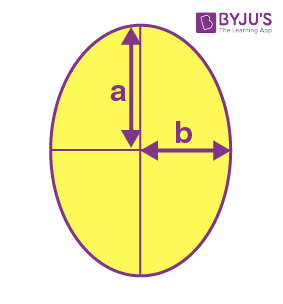Triangle a+b+c 1/2 * b * h —- —-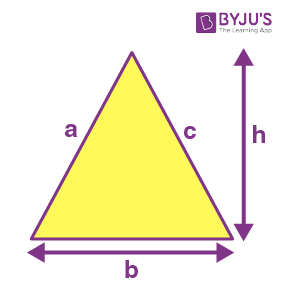Cuboid 4(l+b+h) 2(lb+bh+hl) 2h(l+b) l * b * h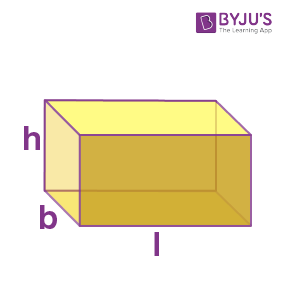Cube 6a 6a2 4a2 a3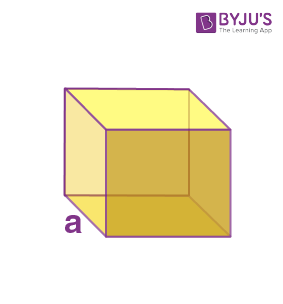Cylinder —- 2 π r(r+h) 2πrh π r2 h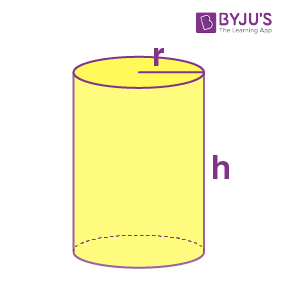Cone —- π r(r+l) π r l 1/3π r2 h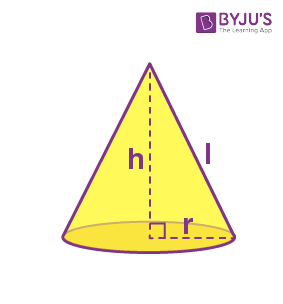Sphere —- 4 π r2 4π r2 4/3π r3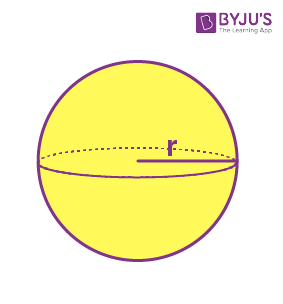Hemisphere —- 3 π r2 2 π r2 2/3π r3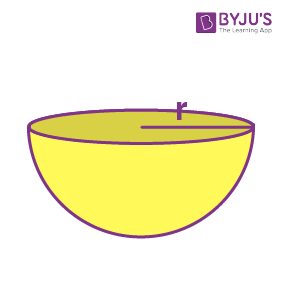## Related Articles

Also have a look on:

## Solved Examples

Example 1:

What is the surface area of a cuboid with length, width and height equal to 4.4 cm, 2.3 cm and 5 cm, respectively?

Solution:

Given, the dimensions of cuboid are:

length, l = 4.4 cm

width, w = 2.3 cm

height, h = 5 cm

Surface area of cuboid = 2(wl+hl+hw)

= 2·(2.3·4.4+5·4.4+5·2.3)

= 87.24 square cm.

Example 2:

What is the volume of a cylinder whose base radii are 2.1 cm and height is 30 cm?

Solution:

Given,

Radius of bases, r = 2.1 cm

Height of cylinder = 30 cm

Volume of cylinder = πr2h = π·(2.1)2·30 ≈ 416.

## Practice Questions on Surface Areas and Volumes

1. Find the volume of a cube whose side length is 5 cm.
2. Find the CSA of the hemisphere, if the radius is 7 cm.
3. If the radius of the sphere is 4 cm, find its surface area.

Stay tuned to BYJU’S – The Learning App and download the app to explore all Maths-related concepts.

## Frequently Asked Questions on Surface Area and Volume

### What are the formulas for surface area and volume of cuboid?

Surface area of cuboid = 2(lb+bh+hl)
Volume = l × b × h
where l = length, b=breadth and h = height.

### What is the total surface area of the cylinder?

The total surface area of the cylinder = 2 π r(r+h), where r is the radius of the circular base and h is the height of the cylinder.

### How to calculate the volume of a cone-shaped object?

If r is the radius of the circular base of the cone-shaped object and h is the height, then the formula to find the volume of the cone is given by: V = 1/3π r2 h

### What is the total surface area of the hemisphere?

The total surface area of the hemisphere is equal to the sum of half of the surface area of sphere and the area of its circular base.
Total surface area of hemisphere = 2 π r2+ π r2 = 3 π r2

Quiz on Surface areas and volumes

1. souvik

You can get all surface area formulas here!
https://byjus.com/surface-area-formulas/

2. its good i uhder stood after seeing this

3. Akshaya Goulikar

Thanks this helped me a lot. So😇😇😇😇

4. NISHANTH

very thanks, it’s very usefull to mee

5. Sharvari dhawale

It’s very useful &helpful for me
Thanks byjus💞🙏

6. Kripa Rai

Thanks a lots 🙂🙂🙂
it’s very useful for me and everyone.
Thank you BYJUS🙏🙏

7. Asisha R Godwin

Thank you so much Byju’s 🤗🤗👍👍it’s so helpful. Thanks a lot.

8. Franklin

Thanks a lot for sharing this info

9. Nishchay Aggarwal

Thanks a lots 🙂🙂🙂
it’s very useful for me and everyone.
Thank you BYJUS🙏🙏

10. anirudh

its good
you can find surface area formulas here

11. Vaishnavi

Thiks alot
It’s very useful for me and everyone
Thinks BYJUS